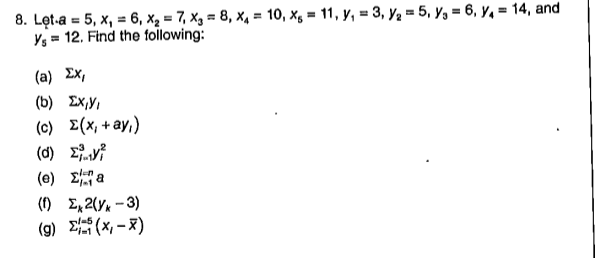# 8. Lęt-a = 5, x, = 6, x, = 7, Xg = 8, x4 = 10, xg = 11, y, = 3, y2 = 5, y, = 6, y, = 14, andYs = 12. Find the following:%3D%3D%3D(a) Ex,(b) Ex,Y,(c) E(x, +ay,)(0) Σ(e) 2 a(1) E, 2(Y* - 3)(g) E(X - x)

Question
1 views

could you help me solve g?help_outlineImage Transcriptionclose8. Lęt-a = 5, x, = 6, x, = 7, Xg = 8, x4 = 10, xg = 11, y, = 3, y2 = 5, y, = 6, y, = 14, and Ys = 12. Find the following: %3D %3D %3D (a) Ex, (b) Ex,Y, (c) E(x, +ay,) (0) Σ (e) 2 a (1) E, 2(Y* - 3) (g) E(X - x) fullscreen
check_circle

Step 1

g.

The sample mean is calculated a...

### Want to see the full answer?

See Solution

#### Want to see this answer and more?

Solutions are written by subject experts who are available 24/7. Questions are typically answered within 1 hour.*

See Solution
*Response times may vary by subject and question.
Tagged in

### Statistics